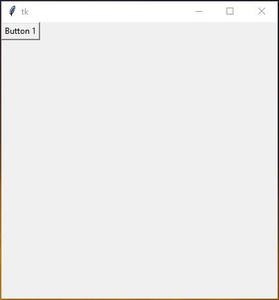Related Articles

# How To Dynamically Resize Button Text in Tkinter?

• Last Updated : 16 Oct, 2021

In this article, we will see how to make the button text size dynamic. Dynamic means whenever button size will change, the button text size will also change. In Tkinter there is no in-built function, that will change the button text size dynamically.

Attention geek! Strengthen your foundations with the Python Programming Foundation Course and learn the basics.

To begin with, your interview preparations Enhance your Data Structures concepts with the Python DS Course. And to begin with your Machine Learning Journey, join the Machine Learning - Basic Level Course

Approach:

• Create button and set sticky to all direction
• Set bind, what bind will do, whenever button size change it will call resize function that we will create later.
• Inside the resize function, we will have a different condition, depends on the main window geometry/size.
• Set row and column configure

Let’s understand with step-by-step implementation:

Step 1: Creates a normal Tkinter window.

## Python3

 `# Import module``from` `tkinter ``import` `*``  ` `# Create object``root ``=` `Tk()``  ` `# Adjust size``root.geometry(``"400x400"``)``  ` `# Execute tkinter``root.mainloop()`

Output:Step 2: Create a button inside the main window.

## Python3

 `# Import module``from` `tkinter ``import` `*` `# Create object``root ``=` `Tk()` `# Adjust size``root.geometry(``"400x400"``)` `# Create Buttons``button_1 ``=` `Button(root , text ``=` `"Button 1"``)` `# Set grid``button_1.grid(row ``=` `0``,column ``=` `0``)` `# Execute tkinter``root.mainloop()`

Output:Step 3: Resizing the button text size

Inside the resize function, the “e” value will tell the main window width and height.

## Python3

 `# resize button text size``def` `resize(e):``    ` `    ``# get window width``    ``size ``=` `e.width``/``10` `    ``# define text size on different condition` `    ``# if window height is greater``    ``# than 300 and less than 400 (set font size 40)``    ``if` `e.height <``=` `400` `and` `e.height > ``300``:``        ``button_1.config(font ``=` `(``"Helvetica"``, ``40``))` `    ``# if window height is greater than``    ``# 200 and less than 300 (set font size 30)``    ``elif` `e.height < ``300` `and` `e.height > ``200``:``        ``button_1.config(font ``=` `(``"Helvetica"``, ``30``))` `    ``# if window height is less than 200 (set font size 40)``    ``elif` `e.height < ``200``:``        ``button_1.config(font ``=` `(``"Helvetica"``, ``40``))`

Below is the full implementation:

## Python3

 `# Import module``from` `tkinter ``import` `*` `# Create object``root ``=` `Tk()` `# Adjust size``root.geometry(``"400x400"``)` `# Specify Grid``Grid.columnconfigure(root, index ``=` `0``,``                     ``weight ``=` `1``)` `Grid.rowconfigure(root, ``0``,``                  ``weight ``=` `1``)` `# Create Buttons``button_1 ``=` `Button(root, text ``=` `"Button 1"``)` `# Set grid``button_1.grid(row ``=` `0``,``              ``column ``=` `0``, sticky ``=` `"NSEW"``)` `# resize button text size``def` `resize(e):``    ` `    ``# get window width``    ``size ``=` `e.width``/``10` `    ``# define text size on different condition` `    ``# if window height is greater``    ``# than 300 and less than 400 (set font size 40)``    ``if` `e.height <``=` `400` `and` `e.height > ``300``:``        ``button_1.config(font ``=` `(``"Helvetica"``, ``40``))` `    ``# if window height is greater than``    ``# 200 and less than 300 (set font size 30)``    ``elif` `e.height < ``300` `and` `e.height > ``200``:``        ``button_1.config(font ``=` `(``"Helvetica"``, ``30``))` `    ``# if window height is less``    ``# than 200 (set font size 40)``    ``elif` `e.height < ``200``:``        ``button_1.config(font ``=` `(``"Helvetica"``, ``40``))` `# it will call resize function``# when window size will change``root.bind(``''``, resize)` `# Execute tkinter``root.mainloop()`

Output:

My Personal Notes arrow_drop_up